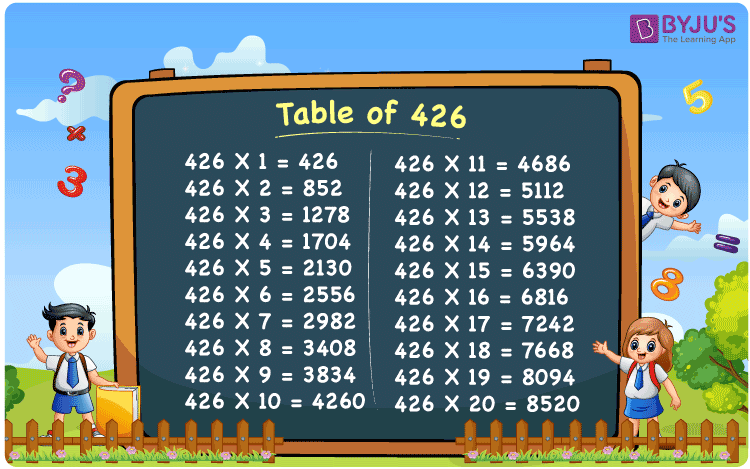Checkout JEE MAINS 2022 Question Paper Analysis : Checkout JEE MAINS 2022 Question Paper Analysis :

# Table of 426

The Table of 426 is provided here up to 20 times. Students can quickly check the multiples of 426 presented in a tabular form. They can also download PDF format of the multiplication table of 426 up to 20, which will benefit them while learning the 426 times table.

## Table of 426 Chart

The table of 426 chart is given here to assist students reviewing the multiples of 426 up to 20. The image of the table of 426 can be used as a flashcard while memorising the multiplication table of 426.## What is the 426 Times Table?

The 426 times table lists multiples of 426, which is the multiplication of any natural number with 426. The table of 426 can also be represented as the repeated addition of 426. The 426 times table is given here both in multiplication and addition form.

 426×1 = 426 426 426×2 = 852 426 + 426 = 852 426×3 = 1278 426 + 426 + 426 = 1278 426×4 = 1704 426 + 426 + 426 + 426 = 1704 426×5 = 2130 426 + 426 + 426 + 426 + 426 = 2130 426×6 = 2556 426 + 426 + 426 + 426 + 426 + 426 = 2556 426×7 = 2982 426 + 426 + 426 + 426 + 426 + 426 + 426 = 2982 426×8 = 3408 426 + 426 + 426 + 426 + 426 + 426 + 426 + 426 = 3408 426×9 = 3834 426 + 426 + 426 + 426 + 426 + 426 + 426 + 426 + 426 = 3834 426×10 = 4260 426 + 426 + 426 + 426 + 426 + 426 + 426 + 426 + 426 + 426 = 4260

## Multiplication Table of 426

Given below is the multiplication table of 426 up to 20.

 426 × 1 = 426 426 × 2 = 852 426 × 3 = 1278 426 × 4 = 1704 426 × 5 = 2130 426 × 6 = 2556 426 × 7 = 2982 426 × 8 = 3408 426 × 9 = 3834 426 × 10 = 4260 426 × 11 = 4686 426 × 12 = 5112 426 × 13 = 5538 426 × 14 = 5964 426 × 15 = 6390 426 × 16 = 6816 426 × 17 = 7242 426 × 18 = 7668 426 × 19 = 8094 426 × 20 = 8520

## Solved Example on the Table of 426

Example 1:

What is 48 times 426?

Solution:

Using the multiplication tables of 426,

426 × 48 = 426 × ( 50 – 2) = (426 × 50) – (426 × 2)

= 21300 – 852 = 20,448.

## Frequently Asked Questions on Table of 426

### What is the times table of 426?

The times table of 426 is the list of multiples of 426 starting from 426 × 1 = 426, 426 × 2 = 852, 426 × 3 = 1278, 426 × 4 = 1704, 426 × 5 = 2130 and so on.

### Which times table has 426?

426 comes in the times tables of 1, 2, 3, 6, 71, 142, 213 and 426.

### What is 4 times 426?

4 times 426 is 1704.